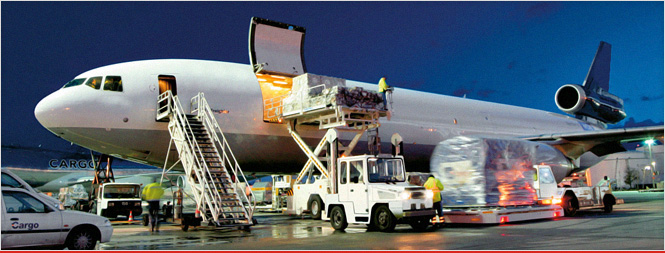# Air Freight / Cargo Calculator

Air Freight costs are determined by Chargeable Weight. The Chargeable Weight (cw) of an airfreight is determined by the actual gross weight (gw) or the volume weight (vw), whichever is greater.Air Freight Chargeable Weight formula:

• Imperial: L x W x H in inches (in.) / 165 = vw (in lbs.)
• Metric: L x W x H in centimetres (cm.) / 6,000 = vw (in kgs)

Air Freight Measurements:

• 6000 cubic centimetres per kilograms
• 366 cubic inches per kilogram
• 166 cubic inches per pound

Examples of Chargeable Weight (cw) Calculations:

Example 1:
Chargeable weight calculation for one (1) pallet load with measurements of 100 cm x 100 cm x 100 cm with a gross weight of 100 kgs

Actual Volume = 1 x 1 x 1 = 1 cubic metre

Calculating Chargeable Weight for 1 cubic metre = 1 /0.006 = 167 kgs (rounded up) and not the gross weight of 100 kgs as volume weight of 167 kgs is the greater.
Example 2:
If using the same measurements for pallet load as above but with an actual gross weight of 200 kgs, the chargeable weight is then 200 kgs as it is greater then the calculated volume weight.